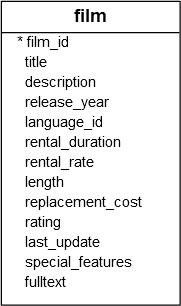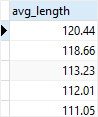# PostgreSQL Tutorial: ALL Operator

August 3, 2023

Summary: in this tutorial, you will learn how to use the PostgreSQL ALL operator to compare a value with a list of values returned by a subquery.

## Overview of the PostgreSQL ALL operator

The PostgreSQL ALL operator allows you to query data by comparing a value with a list of values returned by a subquery.

The following illustrates the syntax of the ALL operator:

``````comparison_operator ALL (subquery)
``````

In this syntax:

• The ALL operator must be preceded by a comparison operator such as equal (=), not equal (!=), greater than (>), greater than or equal to (>=), less than (<), and less than or equal to (<=).
• The ALL operator must be followed by a subquery which also must be surrounded by the parentheses.

With the assumption that the subquery returns some rows, the ALL operator works as follows:

1. `column_name > ALL (subquery)` the expression evaluates to true if a value is greater than the biggest value returned by the subquery.
2. `column_name >= ALL (subquery)` the expression evaluates to true if a value is greater than or equal to the biggest value returned by the subquery.
3. `column_name < ALL (subquery)` the expression evaluates to true if a value is less than the smallest value returned by the subquery.
4. `column_name <= ALL (subquery)` the expression evaluates to true if a value is less than or equal to the smallest value returned by the subquery.
5. `column_name = ALL (subquery)` the expression evaluates to true if a value is equal to any value returned by the subquery.
6. `column_name != ALL (subquery)` the expression evaluates to true if a value is not equal to any value returned by the subquery.

In case the subquery returns no row, then the ALL operator always evaluates to true.

## PostgreSQL ALL operator examples

Let’s use the `film` table from the sample database for the demonstration.The following query returns the average lengths of all films grouped by film rating:

``````SELECT
ROUND(AVG(length), 2) avg_length
FROM
film
GROUP BY
rating
ORDER BY
avg_length DESC;
``````To find all films whose lengths are greater than the list of the average lengths above, you use the ALL and greater than operator (>) as follows:

``````SELECT
film_id,
title,
length
FROM
film
WHERE
length > ALL (
SELECT
ROUND(AVG (length),2)
FROM
film
GROUP BY
rating
)
ORDER BY
length;
``````As you can see clearly from the output, the query return all films whose lengths are greater than the biggest value in the average length list returned by the subquery.

In this tutorial, you have learned how to used the PostgreSQL ALL operator to compare a value with a list of values returned by a subquery.